# tberrios2005 banned for Xraying

Pretty obvious mining pattern. Oh, yeah please stop throwing around diamonds when you Xray, it becomes a bit too obvious.

Spoiler
[Sun Aug 19 00:10:07 HKT 2012] DIAMOND_ORE broken by tberrios2005 at (x=  -295, y=   4, z=  -107, l= 7)
[Sun Aug 19 00:10:12 HKT 2012] DIAMOND_ORE broken by tberrios2005 at (x=  -295, y=   4, z=  -108, l= 8)
[Sun Aug 19 00:10:12 HKT 2012] DIAMOND_ORE broken by tberrios2005 at (x=  -296, y=   4, z=  -108, l= 7)
[Sun Aug 19 00:10:12 HKT 2012] DIAMOND_ORE broken by tberrios2005 at (x=  -296, y=   4, z=  -107, l= 6)
[Sun Aug 19 00:10:17 HKT 2012] DIAMOND_ORE broken by tberrios2005 at (x=  -296, y=   3, z=  -108, l= 6)
[Sun Aug 19 00:10:17 HKT 2012] DIAMOND_ORE broken by tberrios2005 at (x=  -296, y=   3, z=  -107, l= 5)
[Sun Aug 19 00:14:31 HKT 2012] DIAMOND_ORE broken by tberrios2005 at (x=  -295, y=  11, z=   -62, l=11) [t= 253sec / (d= 46 / b= 128) = r=708.4]
[Sun Aug 19 00:14:31 HKT 2012] DIAMOND_ORE broken by tberrios2005 at (x=  -294, y=  11, z=   -62, l=10)
[Sun Aug 19 00:14:31 HKT 2012] DIAMOND_ORE broken by tberrios2005 at (x=  -294, y=  10, z=   -62, l= 9)
[Sun Aug 19 00:14:31 HKT 2012] DIAMOND_ORE broken by tberrios2005 at (x=  -295, y=  10, z=   -62, l=10)
[Sun Aug 19 00:14:37 HKT 2012] DIAMOND_ORE broken by tberrios2005 at (x=  -295, y=  11, z=   -61, l=10)
[Sun Aug 19 00:14:37 HKT 2012] DIAMOND_ORE broken by tberrios2005 at (x=  -295, y=  10, z=   -61, l= 9)
[Sun Aug 19 00:14:52 HKT 2012] DIAMOND_ORE broken by tberrios2005 at (x=  -300, y=   8, z=   -61, l= 2) [t=   0sec / (d=  5 / b=  11) = r=30.6] [flagged x1; ratio paranoidDiamonds]
[Sun Aug 19 00:14:52 HKT 2012] DIAMOND_ORE broken by tberrios2005 at (x=  -300, y=   7, z=   -62, l= 0)
[Sun Aug 19 00:14:57 HKT 2012] DIAMOND_ORE broken by tberrios2005 at (x=  -300, y=   8, z=   -62, l= 1)
[Sun Aug 19 00:14:57 HKT 2012] DIAMOND_ORE broken by tberrios2005 at (x=  -299, y=   7, z=   -62, l= 1)
[Sun Aug 19 00:14:57 HKT 2012] DIAMOND_ORE broken by tberrios2005 at (x=  -299, y=   7, z=   -61, l= 2)
[Sun Aug 19 00:17:08 HKT 2012] DIAMOND_ORE broken by tberrios2005 at (x=  -273, y=  11, z=   -65, l=10) [t= 126sec / (d= 27 / b=  75) = r=355.2]
[Sun Aug 19 00:17:08 HKT 2012] DIAMOND_ORE broken by tberrios2005 at (x=  -273, y=  10, z=   -65, l= 9)
[Sun Aug 19 00:17:08 HKT 2012] DIAMOND_ORE broken by tberrios2005 at (x=  -272, y=  10, z=   -65, l=10)
[Sun Aug 19 00:17:08 HKT 2012] DIAMOND_ORE broken by tberrios2005 at (x=  -272, y=  10, z=   -64, l=11)
[Sun Aug 19 00:17:08 HKT 2012] DIAMOND_ORE broken by tberrios2005 at (x=  -273, y=  10, z=   -64, l=10)
[Sun Aug 19 00:17:40 HKT 2012] DIAMOND_ORE broken by tberrios2005 at (x=  -274, y=  10, z=   -43, l=11) [t=  30sec / (d= 21 / b=  30) = r=42.8] [flagged x2; ratio paranoidDiamonds]
[Sun Aug 19 00:17:40 HKT 2012] DIAMOND_ORE broken by tberrios2005 at (x=  -274, y=   9, z=   -43, l=10)
[Sun Aug 19 00:17:40 HKT 2012] DIAMOND_ORE broken by tberrios2005 at (x=  -274, y=  10, z=   -42, l=10)
[Sun Aug 19 00:17:40 HKT 2012] DIAMOND_ORE broken by tberrios2005 at (x=  -274, y=   9, z=   -42, l= 9)
[Sun Aug 19 00:17:40 HKT 2012] DIAMOND_ORE broken by tberrios2005 at (x=  -275, y=  10, z=   -43, l=10)
[Sun Aug 19 00:17:40 HKT 2012] DIAMOND_ORE broken by tberrios2005 at (x=  -275, y=   9, z=   -43, l= 9)
[Sun Aug 19 00:17:40 HKT 2012] DIAMOND_ORE broken by tberrios2005 at (x=  -275, y=  10, z=   -42, l= 9)
[Sun Aug 19 00:17:46 HKT 2012] DIAMOND_ORE broken by tberrios2005 at (x=  -275, y=   9, z=   -42, l= 8)
[Sun Aug 19 00:20:59 HKT 2012] DIAMOND_ORE broken by tberrios2005 at (x=  -320, y=  14, z=   -53, l= 4) [t= 196sec / (d= 47 / b=  56) = r=235.6] [flagged x3; ratio]
[Sun Aug 19 00:20:59 HKT 2012] DIAMOND_ORE broken by tberrios2005 at (x=  -320, y=  13, z=   -53, l= 4)
[Sun Aug 19 00:21:05 HKT 2012] DIAMOND_ORE broken by tberrios2005 at (x=  -321, y=  13, z=   -53, l= 4)
[Sun Aug 19 00:21:05 HKT 2012] DIAMOND_ORE broken by tberrios2005 at (x=  -321, y=  13, z=   -54, l= 3)
[Sun Aug 19 00:21:05 HKT 2012] DIAMOND_ORE broken by tberrios2005 at (x=  -320, y=  13, z=   -54, l= 4)
[Sun Aug 19 00:22:29 HKT 2012] DIAMOND_ORE broken by tberrios2005 at (x=  -343, y=  10, z=   -30, l= 0) [t=  85sec / (d= 33 / b= 111) = r=282.7]
[Sun Aug 19 00:22:29 HKT 2012] DIAMOND_ORE broken by tberrios2005 at (x=  -344, y=  10, z=   -30, l= 0)
[Sun Aug 19 00:22:34 HKT 2012] DIAMOND_ORE broken by tberrios2005 at (x=  -344, y=   9, z=   -31, l= 0)
[Sun Aug 19 00:22:34 HKT 2012] DIAMOND_ORE broken by tberrios2005 at (x=  -344, y=   8, z=   -31, l= 0)
[Sun Aug 19 00:22:47 HKT 2012] DIAMOND_ORE broken by tberrios2005 at (x=  -345, y=   9, z=   -23, l= 5) [t=   0sec / (d=  8 / b=  17) = r=29.3] [flagged x4; ratio paranoidDiamonds]
[Sun Aug 19 00:22:52 HKT 2012] DIAMOND_ORE broken by tberrios2005 at (x=  -346, y=   7, z=   -22, l= 1)
[Sun Aug 19 00:23:09 HKT 2012] DIAMOND_ORE broken by tberrios2005 at (x=  -342, y=  14, z=   -25, l= 9) [t=   0sec / (d=  9 / b=   8) = r=16.7] [flagged x5; ratio paranoidDiamonds]
[Sun Aug 19 00:23:09 HKT 2012] DIAMOND_ORE broken by tberrios2005 at (x=  -341, y=  14, z=   -25, l= 8)
[Sun Aug 19 00:23:09 HKT 2012] DIAMOND_ORE broken by tberrios2005 at (x=  -342, y=  15, z=   -25, l= 8)
[Sun Aug 19 00:23:09 HKT 2012] DIAMOND_ORE broken by tberrios2005 at (x=  -341, y=  15, z=   -25, l= 7)
[Sun Aug 19 00:23:15 HKT 2012] DIAMOND_ORE broken by tberrios2005 at (x=  -342, y=  14, z=   -24, l= 8)
[Sun Aug 19 00:23:15 HKT 2012] DIAMOND_ORE broken by tberrios2005 at (x=  -341, y=  14, z=   -24, l= 9)
[Sun Aug 19 00:23:15 HKT 2012] DIAMOND_ORE broken by tberrios2005 at (x=  -341, y=  15, z=   -24, l= 8)
[Sun Aug 19 00:23:15 HKT 2012] DIAMOND_ORE broken by tberrios2005 at (x=  -342, y=  15, z=   -24, l= 7)
[Sun Aug 19 00:24:09 HKT 2012] GOLD_ORE    broken by tberrios2005 at (x=  -325, y=   9, z=   -59, l= 9) [t=  51sec / (d= 39 / b=  36) = r=46.6] [flagged x6; ratio]
[Sun Aug 19 00:24:09 HKT 2012] GOLD_ORE    broken by tberrios2005 at (x=  -324, y=   9, z=   -59, l=10)
[Sun Aug 19 00:24:15 HKT 2012] DIAMOND_ORE broken by tberrios2005 at (x=  -327, y=   8, z=   -60, l= 5) [t=   0sec / (d=  3 / b=   8) = r=64.7] [flagged x7; ratio paranoidDiamonds]
[Sun Aug 19 00:24:20 HKT 2012] DIAMOND_ORE broken by tberrios2005 at (x=  -328, y=   8, z=   -60, l= 4)
This entry was posted in Bans by uncovery. Bookmark the permalink.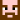Owner & admin of this server.

## 17 thoughts on “tberrios2005 banned for Xraying”

1.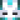skilletfk on said:

2. It’s even more sad considering the time he had been on the server. 567 days is quite a lot tot throw away.

3.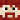psiber on said:

agree on this one. Sad way to lose a long term player.

What a foolish thing to do.

4. Well i guess some people just don’t learn.

5. Really…. 2nd member of my fam get themselves banned? Oh and good news… My house is 3 houses away from him. Next time I visit back home I’m going to pay a visit to him…

• I believe I read an article some time ago about something the scientists called the ‘x-rayers gene.’ Maybe we should get you tested too!

•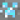Daiyamondo on said:

That might explain that air block house you got!

• Hope i’m not the 3rd member of Hiosa’s family to get banned!!!! XD

•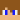kidcodiwise on said:

-FireGuy12 banned by association!-

6. Because i have been mine stripping my mine and i actually didn’t mine any of my diamond (except a few of them), till i got a fortune pickaxe to mine the diamond does that mean that I’m going to be banned??? i understand if the just dig a straight line down and then a straight line to the next diamonds ore, but what happen if you mine strip and then don’t touch the diamonds in a later date?

•uncovery on said:

I do not ban people only from the logfiles. I teleport there and check how it looks like. I can see the strip mines and move on.

•psiber on said:

Heh, bet I raised some flags the other day when i laid down 320 odd silk touched redstone ores onto my skylands lot and busted them all inside a couple mins >.<

7.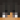tberrios2005 on said:

well guess im joining soulsand45 Dx

8.tberrios2005 on said:

• Kinda stupid to waste over 550 days when you know you’ll get banned for x-raying like many others did.